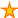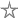# Alternative to Nz for Access v1 / v2

4 votes:3,906 views      No comments
by Allen Browne, 20 April 2005    (for Access v1, v2)

### Null2Zero(), StrLen()

These two functions are very simple but useful in Access 1 and 2, since Nulls propagate like rabbits. From Access 95 onwards, use the built-in Nz() function.

Use Null2Zero() to prevent runtime errors when assigning values to non-variants, or to prevent calculations choking over Nulls.

```Function Null2Zero(AValue)
' Purpose: Return the value 0 if AValue is Null.
If IsNull(AValue) Then
Null2Zero = 0
Else
Null2Zero = AValue
End If
End Function```

Len() returns Null as the length of a Null variant, so StrLen() is a quick substitute. Since any non-zero value = True, you can test for no entry (Null or zero length string) with If Not StrLen([MyControl]) Then

```Function StrLen(AVariant) As Integer
' Returns the length of a variant or string, treating Null as a zero-length string.
If IsNull(AVariant) Then
StrLen = 0
Else
StrLen = Len(AVariant)
End If
End Function```

Rate this article: